华南理工大学 [问题点数：0分]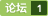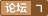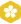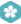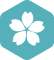2006年11月 Oracle大版内专家分月排行榜第三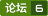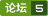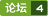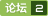2019年华南理工大学程序设计竞赛（春季赛） A NB群友 （记忆化搜索）

<em>华南理工大学</em> 射频电路与天线 之滤波器设计，非常好的设计资料。

1.采用三阶插值的图像任意角度旋转与缩放（DIP 2.4.4, 4.5.4） 2.图像的傅立叶变换，并与功能1联动，输入图像经过旋转、缩放后的傅立叶变换结果可在右侧显示(DIP 4.5, 4.6) 3.给图像添加高斯噪声 (可变参数，DIP 5.2) 4.采用采用平滑线性滤波、高斯滤波、维纳滤波三种方法过滤不同参数的高斯噪声
2013-2014华南理工大学操作系统课程设计（完整源代码和详细文档）
Requirements 1． Simulate a Unix file system on your Windows Platform 2． Understand the file system on Unix system, and the usage of i-nodes 3． Implement the function of sub-directory 4． The task needs to be completed using C++ or C 5． Tasks/Functionalities The following functions are required in your file system: 1． Allocate 16MB space in memory as the storage for your file system. The space is divided as blocks with block size 1KB Assume block address length is 32-bit; Design the information contained in i-node The i-node should support up to 10 direct block addresses The i-node should support at least one indirect block address 2． The fist block is used for storing the i-node for the root directory(/). While your program is lunched, two directories (/dir1 and / dir1/dir2) should be created, and also two files need to be written as /dir1/file1 and /dir1/dir2/file2 (5 marks) 3． File 1 and 2 contain the message of “This is file 1.” and “This is file2”. 4． Following commands should be supported in your system： a) Create a file：createFile fileName fileSize (10 marks) i.e.：createFile /dir1/myFile 1024 (in bytes) if fileSiz > max file size, print out an error message. The file content is filled up with filename + repeated digits from 0 - 9 i.e.: “myFile012345678901234567890123….” b) Delete a file：deleteFile filename (10 marks) i.e.：deleteFile /dir1/myFile c) Create a directory：createDir (5 marks) i.e.：createDir /dir1/sub1 d) Delete a directory：deleteDir (5 marks) i.e.: deleteDir /dir1/sub1 （The current working directory is not allowed to be deleted） e) Change current working direcotry：changeDir (5 marks) i.e.: changeDir /dir2 f) List all the files and sub-directories under current working directory：dir (5 marks) You also need to list at least two file attributes. (i.e. file size, time created, etc.) g) Copy a file : cp (5 marks) i.e.: file1 file2 h) Display the usage of storage space：sum (10 marks) Display the usage of the 16MB space. You need to list how many blocks are used and how many blocks are unused. i) Print out the file contents: cat (10 marks) Print out the contents of the file on the terminal i.e: cat /dir1/file1 5． quit，exit the program and release all the memory occupied；(5 marks) You do not need to save the contents in memory onto hard disk

<em>华南理工大学</em> 信号与系统课件 用的奥本汉姆第二版的教材 考研必备

<em>华南理工大学</em>发布的颜值数据库SCUT-FBP5500，数据集共有5500个人，分值在1~5之间

【C#】华南理工大学计算机考研复试题目
<em>华南理工大学</em>计算机考研复试，在34所408联考高校中比较特别，除去各个学校都存在的面试环节，对比于其它科目传统笔试，<em>华南理工大学</em>更加倾向于使用C#窗体的程序制作来进行面试复试，这与部分学校使用传统的C语言编写算法不同。<em>华南理工大学</em>计算机考研复试的工程量、深度堪比一个小型的软件项目。 网上一直对此资料甚小，有也是部分同学的回忆版，草草地叙述一下题目，也写写简单编程思想。并没有一份详尽的资料。这对于

<em>华南理工大学</em>网络学院-离散数学随堂练习答案
C++作业（华南理工大学网络教育作业)
<em>华南理工大学</em>网络教育作业高级语言程序设计C++•第一次作业 1. 用C++语言编制的源程序要变为目标程序必须要经过( )。 (A) 解释 (B) 汇编 (C) 编辑 (D) 编译 答题： A. B. C. D. 参考答案：D 2. 下列字符列中，合法的标识符是：( ) (A) Begin (B) for (C) file.txt (D) π 答题： A. B. C. D. 参考答案：A 3. 下述叙述正确的是（ ）。 (A)字符型变量不能赋予整数 (B) 任意整数都能表示字符 (C)字符型变量可存放多个字符 (D) 字符数据的值是整数值 答题： A. B. C. D. 参考答案：D 4. 在C++中，char类型数据在内存的存储形式是： (A) 原码 (B) 反码 (C) 补码 (D) ASCII码 答题： A. B. C. D. 参考答案：D 5. 设有 int a,b; 下面（ ）不是C++的正确表达式。 (A)!a (B)b%a (C)a<2 (D)a+b=a 答题： A. B. C. D. 参考答案：D

1、输入一个字符串，要求输出能把所有的小写字符放前面，大写字符放中间，数字放后面，并且中间用空格隔开，如果同种类字符间有不同种类的字符，输出后也要用字符隔开。 例：输入 12abc3KF12 ? ? 输出 abc KF 12 3 12 ? ? 输入 rwr21r3hello666world ? ? 输出 rwr r hello world 21 3 666 代码 #include&amp;...

<em>华南理工大学</em>自动控制原理习题集答案2

2019华南理工大学软件工程考研初试科目、参考书目、招生人数汇总

《计算机控制技术》除较全面介绍简单回路计算机控制系统的构成外，主要围绕采样周期的选择和对象纯滞后时间对系统性能的影响，较系统地介绍相关的计算机控制理论的成果和控制技术。全书内容主要包括计算机控制系统的构成，系统模型，模拟化和直接数字设计法，伺服系统设计法，内模控制算法和自校正控制设计方法，以及部分算法的应用实例共九章。主要章节除有小结，还附有足量的例子和适量的习题。 本书除可作为高等院校工业自动化、计算机应用等专业的教材外，还可供非电类从事计算机控制的研究生和从事计算机控制和自动化工作的工程技术人员参考

<em>华南理工大学</em>计算机作业答案，欢迎下载！

<em>华南理工大学</em> 网络教育 专升本 单片机原理及应用 随堂练习

<em>华南理工大学</em> 通信原理 试卷

<em>华南理工大学</em>有机真题<em>华南理工大学</em>有机真题<em>华南理工大学</em>有机真题<em>华南理工大学</em>有机真题<em>华南理工大学</em>有机真题

<em>华南理工大学</em>网络学院，离散数学，离散数学随堂练习答案

4月4日，<em>华南理工大学</em>发布了该校计算机学院违规更改2018年研究生复试分数问题的处理通报。经查，2018年3月22日，计算机学院2018年硕士研究生招生复试结束后，学院院...

<em>华南理工大学</em>有机真题<em>华南理工大学</em>有机真题<em>华南理工大学</em>有机真题<em>华南理工大学</em>有机真题<em>华南理工大学</em>有机真题<em>华南理工大学</em>有机真题

<em>华南理工大学</em>计算机类专业2017年攻读硕士考试卷，算法语言（C).

1：程序设计题 输入个数不限的数字，以逗号为分隔，以回车键为结束，数字大小在-32768-32767之间。从小到达排序后以4个数每行输出 如： 输入：12,34，-12,23,123,56，-9,789 输出：-12 -9 12 23 34 56 128 789 #include&amp;lt;iostream&amp;gt; #include&amp;lt;string&amp;gt; using nam...
2017年华南理工计算机接口技术随堂练习
2017年华南理工计算机接口技术随堂练习最新版本下载，

<em>华南理工大学</em>考研试题<em>华南理工大学</em>考研试题<em>华南理工大学</em>考研试题<em>华南理工大学</em>考研试题<em>华南理工大学</em>考研试题<em>华南理工大学</em>考研试题

<em>华南理工大学</em>计算方法数值分析实验报告（包括4个实验），需要的同学可以参考下！

<em>华南理工大学</em>《网上学习指南》随堂练习答案（三合一），欢迎下载。

Compiler Construction Experiment 1 Implementing a Scanner for TINY+ You are to write a lexical analyzer/scanner for the language TINY+. Goals 1The input of the scanner is a source code file and the output of the scanner is a stream of tokens. 2Your scanner should go for longest possible match i.e. a string ‘:=’ is to be identified as ‘ass-symbol’ rather than ‘:’ and ‘=’. 3Token is represented as (Kind, Value). We use the following symbols to denote different kinds of tokens KEY denotes reserved words SYM denotes special symbols ID denotes identifiers NUM denotes numeric constants STR denotes string constants 4Check lexical errors: giving meaning error messages and the lines where errors occur. The kinds of lexical errors are: Illegal character, that is, scanner may recognize a character that is not in the alphabet of TINY+, such as \$ is an illegal character The right bracket of a STRING is lost, such as ' scanner The right delimiter of a comment is lost, such as: {this is an example Requirements 1Write your program in C or C++ 2This experiment must be finished in 4 periods. You will submit a report and the source code Example output for some TINY+ programs Test1 or and int bool char while do if then else end repeat until read write , ; := + - * / ( ) < = > = a2c 123 'EFG' The scanner should give the outputs: (KEY, or) (KEY, and) (KEY, int) (KEY, bool) (KEY, char) (KEY, while) (KEY, do) (KEY, if) (KEY, then) (KEY, else) (KEY, end) (KEY, repeat) (KEY, until) (KEY, read) (KEY, write) (SYM, ,) (SYM, ;) (SYM, :=) (SYM, +) (SYM, -) (SYM, *) (SYM, /) (SYM, ( ) (SYM, )) (SYM, ) (SYM, =) (ID, a2c) (NUM, 123) (STR, EFG) Test2 {this is an example} int A,B; bool C1, C2, C3; char D; D:= 'scanner'; while A<=) (ID, B) (KEY, do) (ID, A) (SYM, :=) (ID, A) (SYM, *) (NUM, 2) (KEY, end)

不知不觉在华工已经3年了。    今晚走在回宿舍楼的斜坡上，一时无意识抬头一看：前面横着一栋女生宿舍楼。忽然想到：这个场景，我已经反反复复3年了。但是在意识到3年这个数字之前，却没有一丝丝有很漫长很重复很熟悉的感觉。    （以后补张图）    同样是3年，这跟高中或者初中，给我带来的感受完全是不同的。我现在的手机后置摄像头是坏的，但我一直觉得影响不大：我不是一个念旧的人，不是一个喜欢时刻...

<em>华南理工大学</em>公开课：计算机网络安全 课程1

<em>华南理工大学</em>信号与系统实验 实验五实验报告 优秀

2010-2011华南理工大学操作系统课程设计（完整源代码和详细文档）

c++详细讲解课件（华南理工大学
C++非常详细的讲解，对课程的分析很到位，第一章基本数据与表达式
scut 2018复试机试第一题
<em>华南理工大学</em> 2018 机试第一题，有需要的同学可以下载

<em>华南理工大学</em> 射频电路与天线 之 Microwave office 设计基础资料

#include&lt;cstdio&gt; //将stdio.h的内容用C++的头文件形式表现出来。 #include&lt;algorithm&gt; using namespace std; #define N 100100 int gcd(int a, int b){ return !b ? a : gcd(b, a % b); } int main(){ in...

2012年网上学习指南（一）参考答案，<em>华南理工大学</em>网络教育系列

2019年华南理工大学程序设计竞赛（春季赛）H Parco_Love_GCD （思维 + 数论 + 前缀和）

2019年华南理工大学程序设计竞赛（春季赛）题解

<em>华南理工大学</em>分布式第2章平时作业，需要的童鞋可以下载！

<em>华南理工大学</em>有机真题<em>华南理工大学</em>有机真题

Experiment 1 The QuickSort Algorithm 1.Introduction to the quicksort algorithm In order to sort the input data sequence S, we can do like below: 1)Select one number q and then divide the sequence S into three sub-suquences: S1 in which all of elements are less than q, S2 in which all of elements are equal to q, and S3 in which all of elements are larger than q; 2)Then to sort S1 and S3 with the same algorithm using recursive call itself. 2. Experimental Purposes （1）Learn the sorting algorithms. （2）Understand the difference between the quicksort algorithm and other sorting algorithms, such as: insertion sorting algorithm, straight selection algorithm, etc.. （3）Simulate these algorithms using computer with high-level languages. （4）Solve some sorting problem with different sort algorithms. 3. Abstract of Experiment contents Use QuickSort algorithm to sort the array S that has n elements and constructed by the random() function. To compare the result with ones solved by other sorting algorithms, such as Straight selection sort, insert sort, etc., and understand the difference among them and know how to select some better sorting algorithm while solving some sorting problem. 4. Experimental Requirements 1)The template should be used for all kinds of data type, such as: integer, real, double, etc. in the program; 2)Programs should be made by Object-Oriented Programming (OOP) method; 3)The results should be compared with ones of other algorithms, such as: Straight selection sort, insert sort, Heapsort, etc., and draw the graph to find their differences. Figure 1 The difference between quicksort and insertion sort 4)Write down the report in which there should be the execution results of the program. 5. Example code with C++ ………. void myquicksort(int* A, int l,int r) { if(l>=r) return ; int i=l,j=r; int temp; //Use it to divide A into S1, S2,and S3 ……. //Partition S into S1, S2 and S3 here ……. myquicksort(A,l,i-1); //recursive call for the left part myquicksort(A,i+1,r); //recursive call for the right part } ……………. Experiment 2 The Knapsack Problem Solved by Greedy Algorithm 1.Introduction to the Greedy algorithm (1)Suppose that a problem can be solved by a sequence of decisions. (2)The greedy method has that each decision is locally optimal. (3)These locally optimal solutions will finally add up to a globally optimal solution. 2.Experimental Purposes （1）Understand what is the knapsack problem and the 0/1 knapsack problem; （2）Learn what is the greedy selection strategy and the greedy algorithm; （3）Learn how to solve some optimal problem with greedy algorithm; （4）Compare the greedy algorithm with some other algorithms such as: tree search algorithm. 3. Experimental contents （1）Given a knapsack with the capacity M and some items with its weight and profit, to solve it using Greedy method and Search tree method. （2）Then compare this results with ones of 0/1 Knapsack problem based on the capacity of M. （3）These items could be given likes: •M = 30, •(P1, P2, P3, P4, P5, P6)=(25,24,15,18,22,35) •(W1, W2, W3, W4, W5, W6) = (12, 15, 10, 8, 9, 11) （4）These items could constructed by the ramdom() function in some range, such as: (Wmin, Wmax) and (Pmin, Pmax) and the number of item could be more. 4. Experimental Requirements 1)The template should be used for all kinds of data type, such as: integer, real, double, etc. in the program; 2)Programs should be made by Object-Oriented Programming (OOP) method; 3)Use Greedy method and Search tree method to solve this problem. 4)And compare the results between the knapsack problem and the 0/1 knapsack problem. 5)Write down the report in which there should be the execution results of the program. Experiment 3 The Prim Algorithm for MST 1.Introduction to the Prim Algorithm for MST (1) The definition of Minimum spanning trees (MST): •G = (V, E) is a weighted connected undirected graph; •Spanning tree is S = (V, T), T E, undirected tree; •Minimum spanning tree (MST) is a spanning tree with the smallest total weight. (2) The Prim Algorithm for finding MST: Step 1: x V, Let A = {x}, B = V - {x}. Step 2: Select (u, v) E, u A, v B such that (u, v) has the smallest weight between A and B. Step 3: Put (u, v) in the tree. A = A {v}, B = B - {v} Step 4: If B = , stop; otherwise, go to Step 2. 2. Experimental Purpose (1)Understand what is the Minimum spanning trees (MST); (2)Learn what kinds of algorithms can be used to find MST, such as: Kruskal and Prim algorithms; (3)Compare the difference between these two algorithms; 3. Experimental Contents (1) Given a undirected Graph G=(V, E) like below, to calculate the minimum spanning tree using Kruskal’s algorithm and Prim’s algorithm. 4. Experimental Requirement 1)The template should be used for all kinds of data type, such as: integer, real, double, etc. in the program; 2)Programs should be made by Object-Oriented Programming (OOP) method; 3)Use using Kruskal’s algorithm and Prim’s algorithm to solve this problem. 4)And compare the results between these two algorithms and the difference of selection processes. 5)Write down the report in which there should be the execution results of the program. Experiment 4 The Tree Search Algorithm 1.Introduction to the Tree Search Algorithm The solutions of many problems may be represented by trees and therefore solving them becomes a tree searching problems. We can search this tree to get the solution for this problem. 2.Experimental Purpose （1）Understand the Tree Search algorithm. （2）Learn the solution expression of some problems by A Tree data structure. （3）Understand what kinds of problems can be solved by this algorithm. （4）Realize the source code for this algorithm. （5）Understand different search strategies, such as: the Breadth-first search(BFS) ,the Depth-first search(DFS) and the Best-first search(BFS) Search strategies etc., and compare the difference of results solved by them. 3. Abstract of Experiment contents Given a random initial node with 8 numbers from 1 to 8 and then use the tree search algorithm to find if there is the goal node or not after moving them. Figure 2 The initial node and goal node for the tree search algorithm 4.Experimental Requirements 1)The template should be used for all kinds of data type, such as: integer, real, double, etc. in the program; 2)Programs should be made by Object-Oriented Programming (OOP) method; 3)Use different strategies to perform this algorithm, such as: the Breadth-first search(BFS) ,the Depth-first search(DFS) and the Best-first search(BFS) Search strategies etc.. 4)And compare the result difference solved by them. 5)Write down the report in which there should be the execution results of the program. Experiment 5 A Shortest-Path problem solved by Dynamic programming Algorithm 1.Introduction to the Shortest-Path Problem and Dynamic programming Algorithm 1)Given a set V of vertices and a set E of edges with weights in a multistage undirected graph G=(E,V), please find the shortest path between any two vertices. 2)Dynamic Programming is an algorithm design method that can be used when the solution to a problem may be viewed as the result of a sequence of decisions and this algorithm is a very useful technique to solve many combinatorial optimization problems. In order to solve a problem by using dynamic programming, we should know how to: Find out the recurrence relations. Represent the problem by a multistage graph. 2. Experimental Purpose （1）Know the shortest-path problem for multistage undirected graph can be solved by the dynamic programming algorithm. （2）Learn what is the dynamic programming strategy and the method of this algorithm. （3）Learn how to find out the recurrence relations. （4）Learn how to represent the problem by a multistage graph. 3. Abstract of Experiment contents 1) Given a set V of vertices and a set E of edges with weights in a multistage undirected graph G=(E,V) like below, please find the shortest path between any two vertices using dynamic programming algorithm. Figure 3 The multistage undirected graph G 2) Define a function d(V1, V2): the minimum distance between vertex V1 and V2. 4. Experimental Requirements 1)The template should be used for all kinds of data type, such as: integer, real, double, etc., in the program; 2)Programs should be made by Object-Oriented Programming (OOP) method; 3)Use the backward and forward approaches to perform this algorithm. 4)And compare the efficiency between the backward and forward approaches. 5)Write down the report in which there should be the execution results of the program.

【C#】2015华南理工大学计算机考研复试题目
https://blog.csdn.net/yongh701/article/details/50467129

1.sql约束 where sname like ‘张_’ where sname like ‘张[0-9]’ int num not null check(num&amp;lt;=30) sex char(2) not null check(sex in(‘男’,’女’)) check([EmpSex]=’男’ or [EmpSex]=’女’) 2.DBHelperSQL.cs usi...

-

C++语言详解（华南理工大学
C++非常详细的讲解，对课程的分析很到位，第二章 程序控制结构
2018年华南理工大学824信号与系统考研试题
2018年<em>华南理工大学</em>824信号与系统考研试题，适用专业：电路与系统、信号与信息处理、电磁场、生物医学工程、通信与信息系统

<em>华南理工大学</em> 电路课件 期末考试试题 试卷是03-05年的。

<em>华南理工大学</em>c++ppt课件，内容不错，值得一看。

<em>华南理工大学</em>cisco网络学院CCNA试验手册<em>华南理工大学</em>cisco网络学院CCNA试验手册<em>华南理工大学</em>cisco网络学院CCNA试验手册<em>华南理工大学</em>cisco网络学院CCNA试验手册
C#毕业设计+源码下载